# Properties Of Circle: Download Mathematics Study Notes Free PDF For REET Exam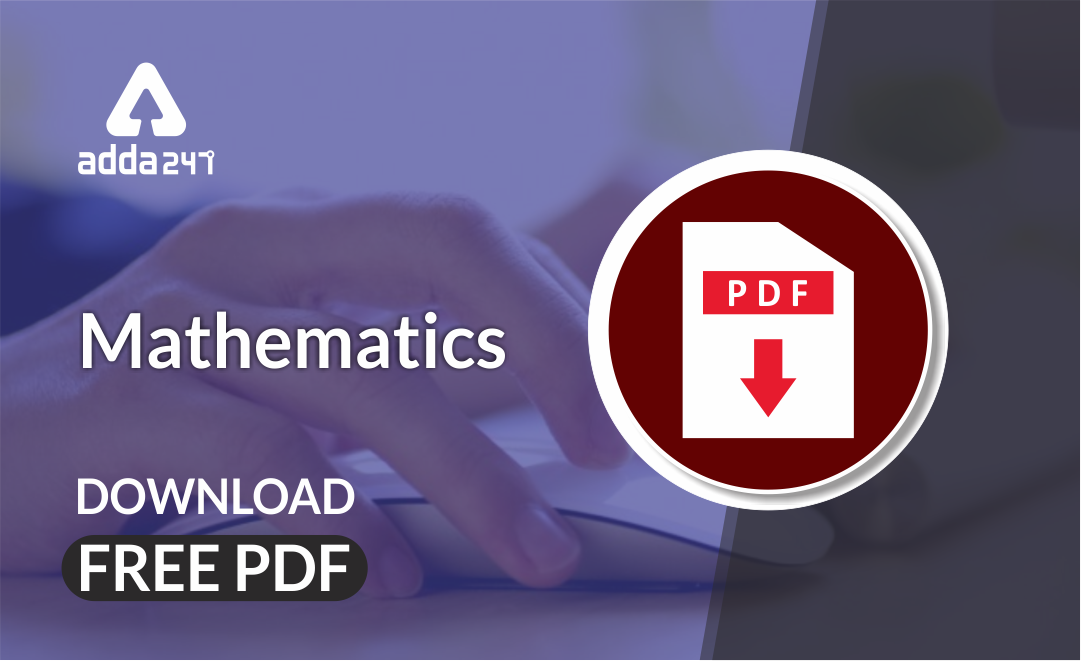Mathematics is an equally important section for  REETMPTET & DSSSB Exams and has even more abundant importance in some other exams conducted by central or state govt. Generally, there are questions asked related to basic concepts and Facts of the Properties of the circles.

To let you make the most of Mathematics section, we are providing important facts related to the Properties of the circles. At least 2-3 questions are asked from this topic in most of the teaching exams. We wish you all the best of luck to come over the fear of the Mathematics section.

How to Overcome Exam Fever, Especially When You Fear Maths

## Properties of Circle

1. A chord which passes through the centre is called the diameter of the circle. It is a largest chord of the circle.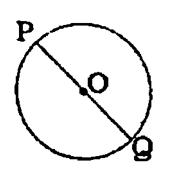1. The perpendicular from the centre of a circle to a chord bisects the chord.

i.e. If OM  AB, then AM = BM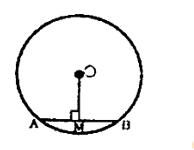Converse of the above theorem: – The line joining the centre of a circle to the midpoint of a chord is perpendicular to the chord.

i.e. AM = MB, then OM  AB.

1. Equal chords of a circle subtend equal angles at the centre.

i.e. If AB = CD, then ∠1 = ∠2.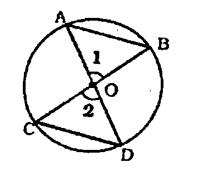Converse of the above theorem: – Angles subtended by two chords at the centre of a circle are equal then the chords are equal.

i.e. If ∠1 = ∠2, then AB = CD.

Get free Study material for REET Exam

1. Equal chords of a circle are equidistance from the centre.

i.e. If AB = CD, OX  AB and OY CD, then OX = OY.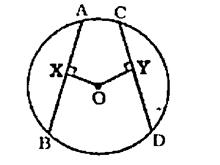Converse of the above theorem:  chords equidistant from the centre of the circle area equal.

i.e. If OX  AB, OY CD and OX = OY then AB = CD.

1. Degree Measure Theorem:

The angle subtended by a chord at the centre of a circle is twice the angle subtended by the chord at any point on the major arc.

i.e. ∠x at the centre and ∠ y at the circumference made by the same arc AB, then ∠x = 2∠y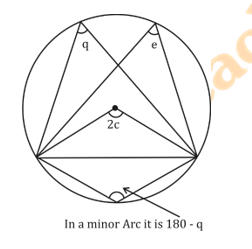1. Angles in the same segment of a circle are equal.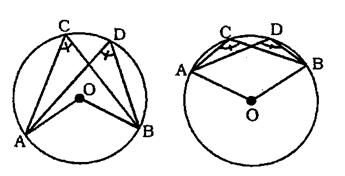(angles in same arc) or (angles in same segment)

Mathematics Study Notes For All Teaching Exams

1. The angles in a semi circle is a right angle.

i.e.∠ ACB = 90°.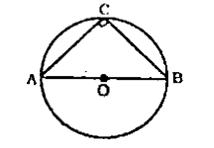Converse of the above theorem: The circle, drawn with hypotenuse of a right triangle as diameter, passes through its opposite vertex.

Practice REET Previous Year Maths Quiz For REET Exam Here

1. If ∠ APB = ∠ AQB, and If P, Q are on the same side of AB, then A, B, Q, O & P are concylic i.e. lie on the same circle.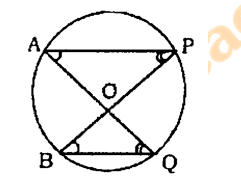1. The sum of the either pair of the opposite angles of a cyclic quadrilateral is 180°.

i.e. ∠ A + ∠ C = ∠ B + ∠ D = 180°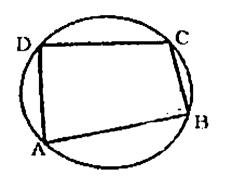•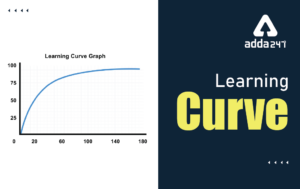Learning Curve - What is Learning Curve?...
•रस - परिभाषा, भे�...
•पठन कौशल- परिभा�...
•अलंकार - परिभाष�...
•Indian States and Capitals 2022: 28 Stat...
•New Education Policy(NEP) PDF - नई �...